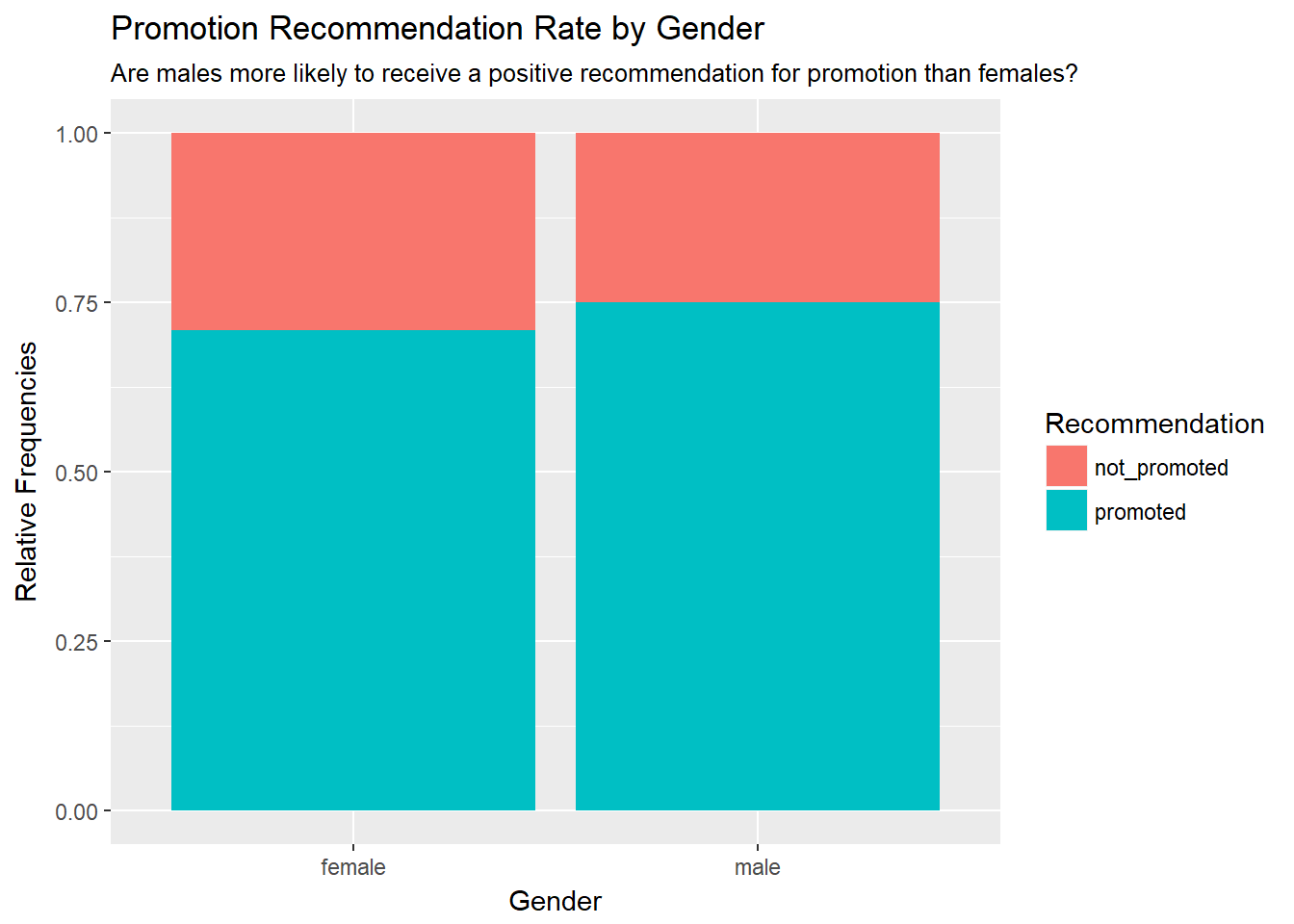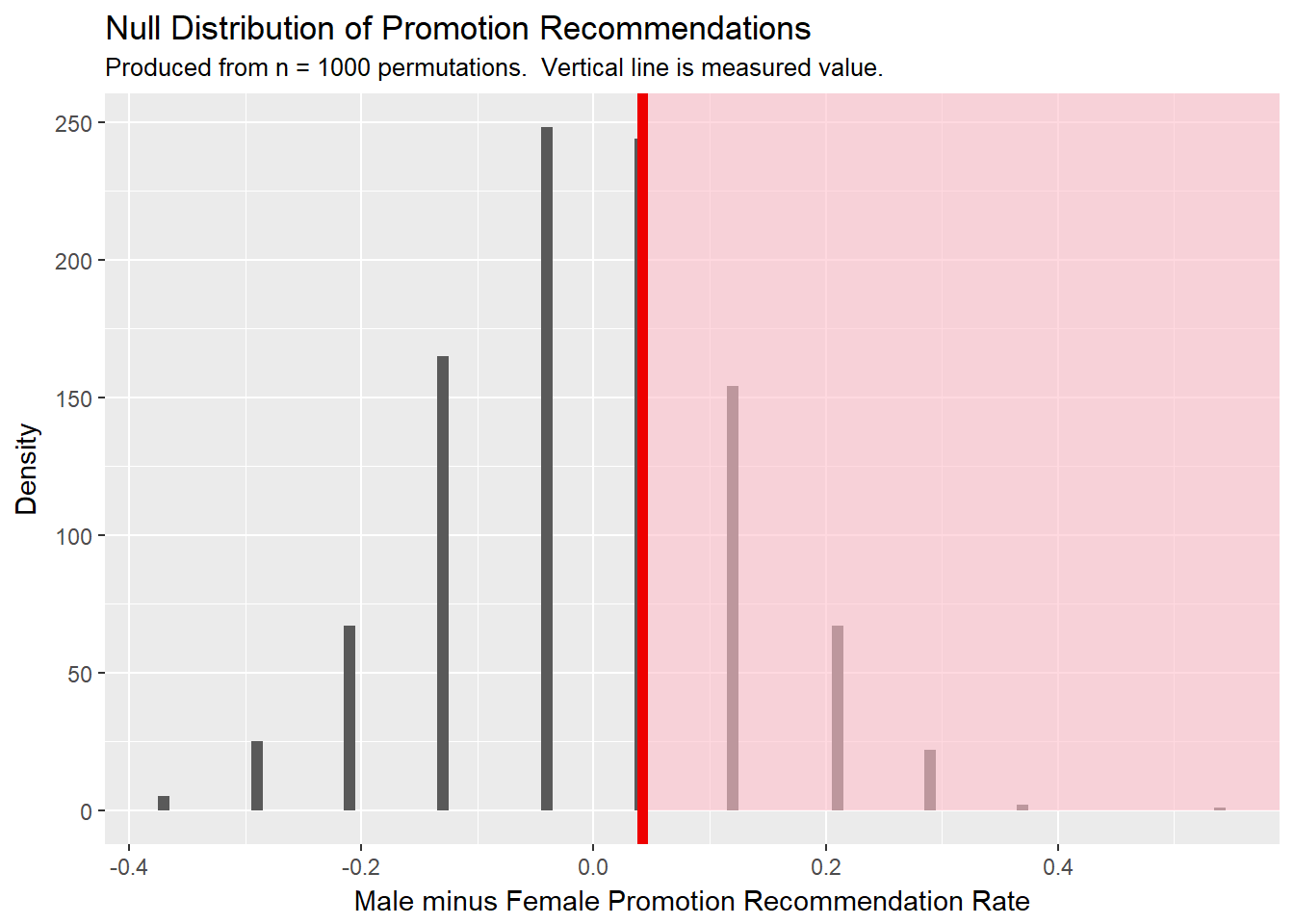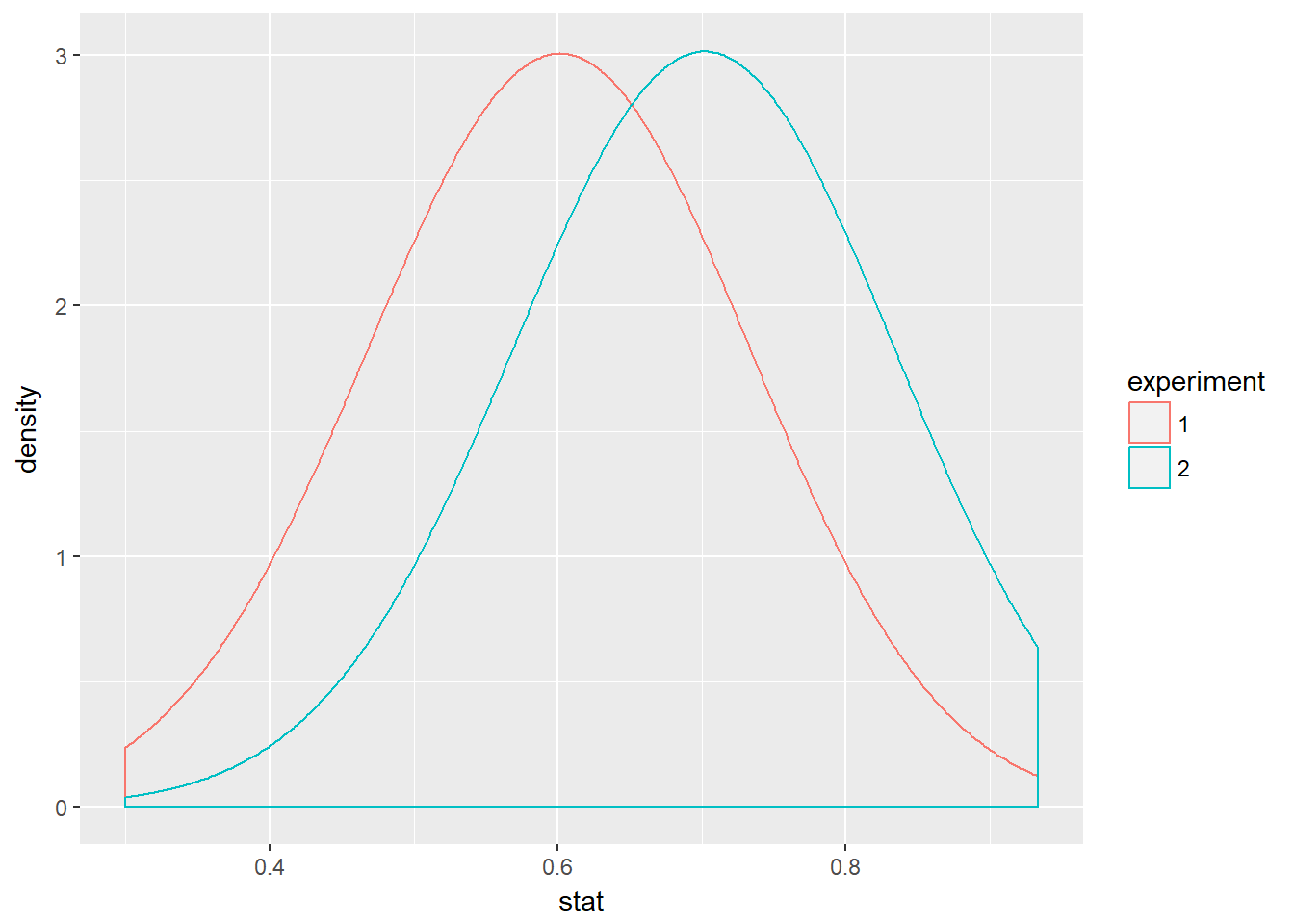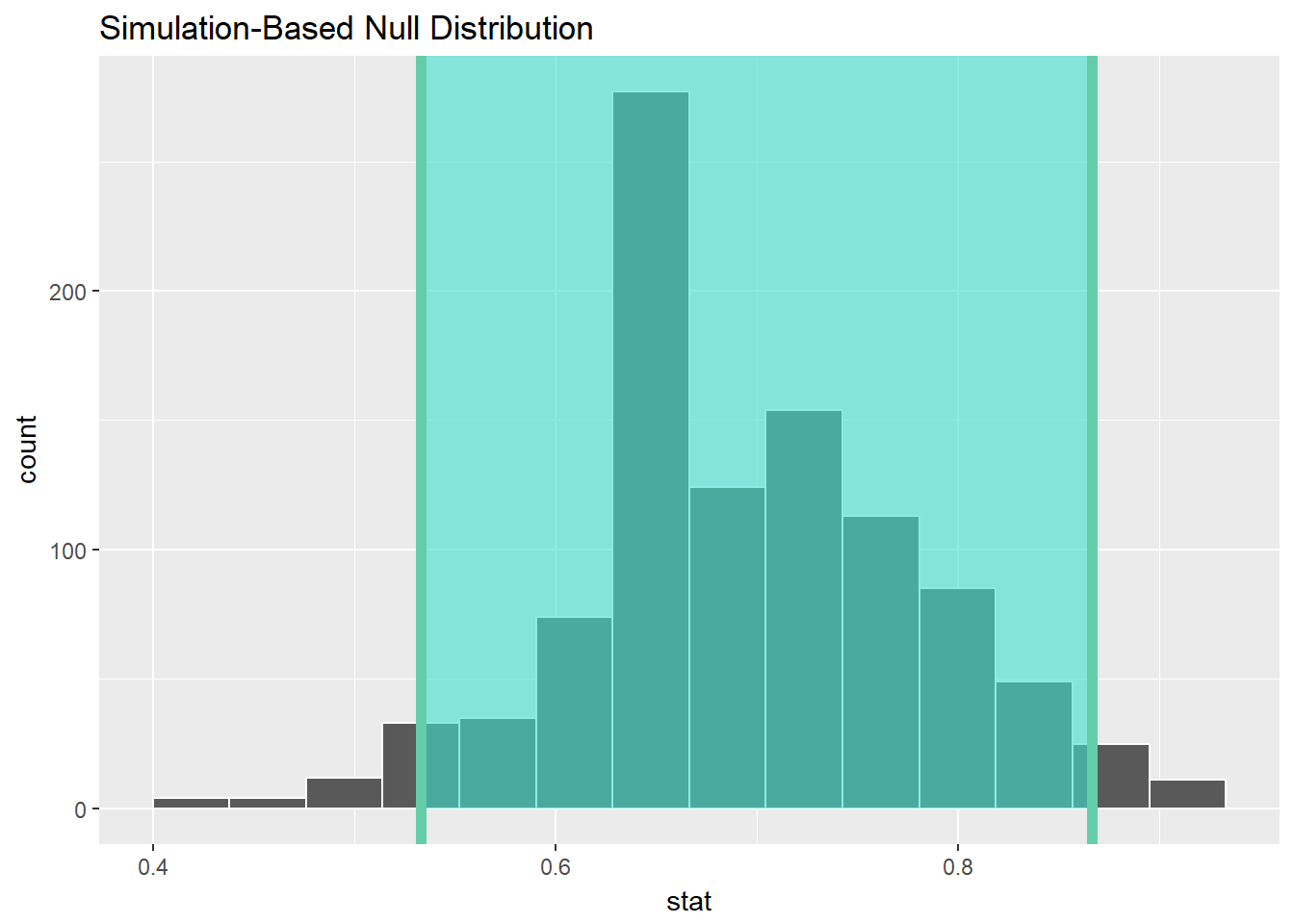My notes from DataCamp course Foundations of Inference.

## Introduction

Statistical inference is the process of making claims about a population based on information from a sample. Inferential statistics attempts to reject a null hypothesis, $$H_0$$. The logic of statistical inference is to compare an observed statistic to the distribution of statistics arising from the null distribution.

## Resources

library(NHANES)
## Warning: package 'NHANES' was built under R version 3.4.4
library(ggplot2)
library(dplyr)
library(infer)
## Warning: package 'infer' was built under R version 3.4.4
disc <- readRDS("Data/disc_new.rds")

## Hypothesis Test

Suppose we are interested in the promotion recommendation rates for males and females. Are resumes with male names more likely to receive a recommendation for promotion than resumes with female names? Our gender discrimination study hypotheses are:
$$H_0$$: gender and promotion are unrelated.
$$H_A$$: men are more likely to be promoted.

# glimpse(disc)

ggplot(disc, aes(x = sex, fill = promote)) +
geom_bar(position = "fill") +
labs(y = "Relative Frequencies",
x = "Gender",
title = "Promotion Recommendation Rate by Gender",
subtitle = "Are males more likely to receive a positive recommendation for promotion than females?",
fill = "Recommendation")# Counts and proportions (as shown in course)
#disc %>%
#  count(promote, sex)
#disc %>%
#  group_by(sex) %>%
#  summarize(promoted_prop = mean(promote == "promoted"))

# Better way: Contingency table
table(disc$promote, disc$sex)
##
##                female male
##   not_promoted      7    6
##   promoted         17   18
options(scipen = 999, digits = 3) # sig digits
# marginal proportion (margin = 2 for cols, 1 for rows)
prop.table(table(disc$promote, disc$sex), margin = 2)
##
##                female  male
##   not_promoted  0.292 0.250
##   promoted      0.708 0.750
# Calculate difference in proportions, male - female
diff_orig <- disc %>%
group_by(sex) %>%
summarize(promoted_prop = mean(promote == "promoted")) %>%
summarize(stat = diff(promoted_prop)) %>%
pull()

Our sample statistic for male promotion rate minus female promotion rate, diff_orig is 0.042. Is this a significant difference, or simply the product of natural variability in the data? We can test this by modeling natural variability, the null distribution. Shuffle observations to remove any relationships that might exist in the population.

Use the infer package to model the null distribution $$H_0$$ of no relationship between promote and sex. 1. Specify the model with specify(y ~ x, success), defining the success condition for the proportion.
2. Set the null hypothesis with hypothesize(null = "independence").
3. Generate permutations of the data with generate(reps = n).
4. Calculate summary statistics with calculate

disc_perm <- disc %>%
specify(promote ~ sex, success = "promoted") %>%
hypothesize(null = "independence") %>%
generate(reps = 1000, type = "permute") %>%
calculate(stat = "diff in props",
order = c("male", "female"))

med_diff <- disc_perm %>%
summarise(med_diff = median(stat)) %>%
pull()

ggplot(disc_perm, aes(x = stat)) +
geom_histogram(binwidth = 0.01) +
geom_vline(aes(xintercept = diff_orig), color = "red") +
labs(y = "Density",
x = "Male minus Female Promotion Recommendation Rate",
title = "Null Distribution of Promotion Recommendations",
subtitle = "Produced from n = 1000 permutations.  Vertical line is measured value.")The observed difference of 0.042 is greater than the density of shuffled differences centered at -0.042. How many randomly permuted differences were as extreme as the observed difference (the shaded area)?

disc_perm %>%
summarize(
q.01 = quantile(stat, p = 0.01),
q.05 = quantile(stat, p = 0.05),
q.10 = quantile(stat, p = 0.10),
q.90 = quantile(stat, p = 0.90),
q.95 = quantile(stat, p = 0.95),
q.99 = quantile(stat, p = 0.99)
)
## # A tibble: 1 x 6
##     q.01   q.05   q.10  q.90  q.95  q.99
##    <dbl>  <dbl>  <dbl> <dbl> <dbl> <dbl>
## 1 -0.292 -0.208 -0.125 0.125 0.208 0.292
disc_perm %>%
summarize(less_extreme = sum(diff_orig > stat),
as_extreme = sum(diff_orig <= stat))
## # A tibble: 1 x 2
##   less_extreme as_extreme
##          <int>      <int>
## 1          510        490
p_value <- disc_perm %>%
get_p_value(obs_stat = diff_orig, direction = "greater") %>%
pull()

The proportion of permutations which produced a difference in rates at least as extreme as the measured value was 0.49, so do not reject $$H_0$$. Our data is consistent with the $$H_0$$.

Note the tradeoff between the two possible errors in hypothesis testing:
A Type I error occurs when one rejects $$H_0$$ when $$H_0$$ is true (convicting an innocent person).
A Type II error occurs when one does not $$H_0$$ when $$H_0$$ is not true (letting a criminal walk).

## Estimation with Confidence Intervals

Whereas the goal of hypothesis testing is to disprove a research claim, the goal of confidence intervals is to estimate a population parameter.

The parameter is a numerical value from the population. The confidence interval is a range that captures the parameter value with a specified degree of confidence. Confidence intervals arise from sampling variability, the variability in sample proportions due to sampling different randomly selected individuals from the population.

Analagous to comparing a value to the null distribution, for confidence intervals we construct a sampling distribution from the population. We can do this with bootstrapping. Bootstrapping is sampling with replacement.

In ex1_props calculate the percent of yes votes from each of 1000 samples from the population. In ex2_props calculate the percent of yes votes from a single sample resampled with replacement 100 times. The standard deviation of the distribution of sample estimates is nearly the same each time. Bootstrapping works as a way of estimating the population sampling distribution!

all_polls <- readRDS("Data/all_polls.rds")

all_polls_samp <- all_polls %>%
group_by(poll) %>%
summarize(stat = mean(vote == "yes"))

one_poll_boot <- all_polls %>%
filter(poll == 1) %>%
select(vote) %>%
specify(response = vote, success = "yes") %>%
generate(reps = 1000, type = "bootstrap") %>%
calculate(stat = "prop")

# Variability of p-hat
all_polls_samp %>%
summarize(variability = sd(stat))
## # A tibble: 1 x 1
##   variability
##         <dbl>
## 1      0.0868
# Variability of p-hat*
one_poll_boot %>%
summarize(variability = sd(stat))
## # A tibble: 1 x 1
##   variability
##         <dbl>
## 1      0.0868
both_props <- bind_rows(all_polls_samp, one_poll_boot, .id = "experiment")
ggplot(both_props, aes(x = stat, color = experiment)) +
geom_density(bw = 0.1)The empircal rule is that if the variability of the sample proportion (standard error, $$SE$$) is known, then approximately 95% of $$\hat{p}$$ values (from different samples) will be within $$2SE$$ of the true population proportion. Or put the other way,

# The true population proportion of yes votes
true_prop_yes <- 0.6

# Proportion of polls within 2SE
all_polls_samp %>%
mutate(is_in_conf_int = abs(stat - true_prop_yes) < 2 * sd(stat)) %>%
summarize(prop_in_conf_int = mean(is_in_conf_int))
## # A tibble: 1 x 1
##   prop_in_conf_int
##              <dbl>
## 1            0.966

Calculate the 95% confidence interval from the bootstrap samples as $$\hat{p}$$ $$\pm 2SE$$.

p_hat <- all_polls %>%
filter(poll == 1) %>%
select(vote) %>%
summarize(stat = mean(vote == "yes")) %>%
pull()

# Create an interval of plausible values
one_poll_boot %>%
summarize(
lower = p_hat - 2*sd(stat),
upper = p_hat + 2*sd(stat)
)
## # A tibble: 1 x 2
##   lower upper
##   <dbl> <dbl>
## 1 0.526 0.874
# Equivalently,
one_poll_boot %>%
summarize(
lower = quantile(stat, p = .025),
upper = quantile(stat, p = .975)
)
## # A tibble: 1 x 2
##   lower upper
##   <dbl> <dbl>
## 1 0.533 0.867
# Or...
percentile_ci <- one_poll_boot %>%
get_confidence_interval(level = 0.95)

one_poll_boot %>%
# Visualize in-between the endpoints given by percentile_ci
visualize(endpoints = percentile_ci, direction = "between")
## Warning: visualize() shouldn't be used to plot confidence interval.
## Arguments endpoints, endpoints_color, and ci_fill are deprecated. Use
## shade_confidence_interval() instead.This analysis was predicated on the assumptions of a sampling distribution that is reasonably symmetric and bell-shaped and a sample size that is reasonably large.

One additional element that changes the width of the confidence interval is the sample parameter value, $$\hat{p}$$. Generally, when the true parameter is close to 0.5, the standard error of $$\hat{p}$$ is larger than when the true parameter is closer to 0 or 1. When calculating a bootstrap t-confidence interval, the standard error controls the width of the CI, and here (given a true parameter of 0.8) the sample proportion is higher than in previous exercises, so the width of the confidence interval will be narrower.